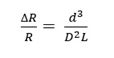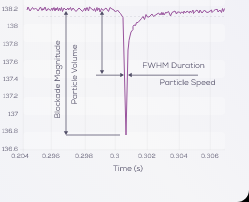# How does the instrument measure particle diameter?

In TRPS, particle volume is proportional to the magnitude of the resistive pulse. Hence, the particle diameter can be determined with extremely high accuracy in a particle-by-particle manner.

Doubling the particle diameter results in an eight fold increase in resistive pulse magnitude, which is why TRPS has very high sensitivity to small differences in particle diameter.

For a spherical particle and a cylindrical pore the simplified relationship is:where R is the pore resistance, D is the pore diameter, d is the particle diameter and L is the effective pore length (which is the geometric length + 0.8 D). This represents the simplest case of a spherical particle and a cylindrical pore. For more complex particle and pore geometries the expression is more involved.

In order to account for different pore geometries and generate accurate and reliable measurements of particle size, the pore is calibrated with a calibration standard of known size. As the software records blockades in nA, calibration particles of known size are used to convert blockade magnitude (nA) into a diameter (nm). This calibrated method ensures accurate and repeatable consistent data across different users and different instruments.

For each sample particle passing through the nanopore: